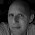Thursday, November 5, 2009

Function._

If you have not looked at the Function object I would recommend you do. It contains several useful methods for combining and converting functions.

Examples:
1. cala> def twoParamFunc( i:Int, j:String) = println(i,j)
2. twoParamFunc: (i: Int,j: String)Unit
3. // the tupled method converts the function with n parameters into
4. // a function that takes one tupleN
5. scala> val tupled = Function tupled twoParamFunc _
6. tupled: ((IntString)) => Unit = < function1>
7. scala> tupled (1 -> "one"
8. (1,one)
9. // A practical use-case is to convert an existing method
10. // for use with a Map
11. // Example with out using the tupled method
12. scala> Map( 1 -> "one", 2 -> "two") map (entry => twoParamFunc(entry._1, entry._2))
13. (1,one)
14. (2,two)
15. res5: scala.collection.immutable.Iterable[Unit] = List((), ())
16. // example using tupled
17. scala> Map( 1 -> "one", 2 -> "two") map (tupled)
18. (1,one)
19. (2,two)
20. res7: scala.collection.immutable.Iterable[Unit] = List((), ())
21. // and for balance the opposite
22. scala> val untupled = Function untupled tupleParamFunc _
23. untupled: (IntString) => Unit = < function2>
24. scala> untupled(1, "one"
25. tuple = (1,one)
26. // Chain is for chaining together an arbitrary number of functions
27. scala> def inc(i:Int) = i + 1
28. inc: (i: Int)Int
29. scala> def double(i:Int) = i*i
30. double: (i: Int)Int
31. scala> def decr(i:Int) = i - 1
32. decr: (i: Int)Int
33. scala> (Function chain List(inc _, double _, decr _, double _, inc _, inc _))(3)
34. res10: Int = 227
35. // Now examples curries two methods then chains them together
36. // define basic methods
37. scala> def inc(step:Int,i:Int) = i + step
38. inc: (step: Int,i: Int)Int
39. scala> def multiply(i:Int, j:Int) = i * j
40. multiply: (i: Int,j: Int)Int
41. // Convert them to methods where the first argument is 3
42. scala> val inc3 = (Function curried inc _)(3)
43. inc3: (Int) => Int = < function1>
44. scala> val multiplyBy3 = (Function curried multiply _)(3)
45. multiplyBy3: (Int) => Int = < function1>
46. // chain the resulting Function1 objects together and execute chain with parameter 3
47. // (3 + 3)*3 = 18
48. scala> chain(List(inc3, multiplyBy3))(3)
49. res12: Int = 18

1 comment:

1.Jesse, just wanted to stop by to say your blog must be one of the best around. By now I stopped counting how many times I was searching for an answer on a Scala topic and found it right here on your blog. Thanks!